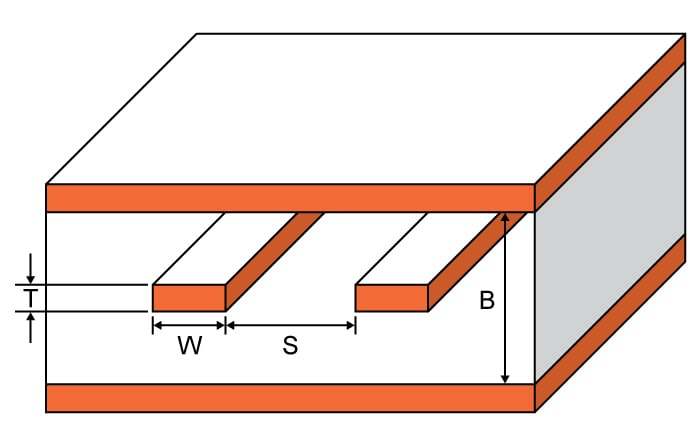# Edge Coupled Stripline Impedance Calculator

## A calculator for computing the impedance of an edge coupled stripline.

Ohms
Ohms
Ohms
Ohms

### Overview

This calculator is designed to calculate the characteristic impedance of an edge coupled stripline. Such a stripline is constructed with two traces referenced to the same reference planes above and below the traces with a dielectric material between them. One of the features of this type of microstrip is the coupling between lines. Matching the differential trace length and keeping the distances between the traces consistent is a common practice.

To use this tool, enter the values for trace thickness, substrate height, trace width, trace spacing and subtrate dielectric in the calculator above and press the "calculate" button. The outputs impedances can be odd, even, common and differential. See below for the definition of these impedances. The default units for all given values, except the subtrate dielectric, is in millimetres. It is possible to select other units.### Equations

$$Z_{0_{ss}}=symmetric\:stripline(w,t,h,er)$$

Where:

$$h=\frac{b-t}{2}$$

$$ke=\tanh \left ( \frac{\pi w}{2b} \right )\cdot \tanh \left ( \frac{\pi }{2}\cdot\frac{w+s}{b} \right )$$

$$ko=\tanh \left ( \frac{\pi w}{2b} \right )\cdot \coth \left ( \frac{\pi }{2}\cdot\frac{w+s}{b} \right )$$

$$k_{e}^{}=\sqrt{1-ke^{2}}$$

$$k_{o}^{}=\sqrt{1-ko^{2}}$$

$$Z_{0_{e}}=\frac{30\pi }{\sqrt{er}}\cdot \frac{K(k_{e}^{})}{K(k_{e})}$$

$$Z_{0_{0}}=\frac{30\pi }{\sqrt{er}}\cdot \frac{K(k_{o}^{})}{K(k_{o})}$$

$$C_{f}^{}\left ( \frac{t}{b} \right )=\frac{.0885\eta_{r}}{\pi }\left \{ \frac{2b}{b-t}\ln \left ( \frac{2b-t}{b-t} \right )-\left ( \frac{t}{b-t} \right )\ln \left ( \frac{b^{2}}{\left ( b-t \right )^{2}}-1 \right ) \right \}$$

$$C_{f}^{}\left ( 0 \right )=\frac{.0885e_{r}}{\pi }\cdot 2\ln \left ( 2 \right )$$

$$k_{ideal}=\text sech\left ( \frac{\pi w}{2b} \right )$$

$$k_{ideal}^{`}=\text tanh\left ( \frac{\pi w}{2b} \right )$$

### Notes:

Odd Impedance ($$Z_{0_{odd}}$$): The impedance between one differential trace and the ground plane with the differential signals are driven with opposite polarity.

$$Z_{0_{odd}}=\frac{Z_{0_{diff}}}{2}$$

Even Impedance ($$ZZ_{0_{even}}$$): The impedance between one differential trace and the ground plane with the differential signals are driven with the same signal.

$$Z_{0_{even}} = 2Z_{0_{common}}$$

Differential Impedance ($$Z_{0_{diff}}$$): The impedance between the two lines with opposite polarity signals.

$$Z_{0_{diff}} = 2Z_{0_{odd}}$$

Common Impedance ($$Z_{0_{common}}$$):The impedance between the two lines with the same signal.

$$Z_{0_{common}}=\frac{Z_{0_{even}}}{2}$$

### Applications

Microwave antennas, couplers and filters can be created using the edge-coupled stripline. These transmission lines are popular because they can be manufactured cheaper than the traditional waveguide and are also more portable. A stripline is easier to construct than the microstrip. The disadvantage of edge-coupled striplines are their limited power-handling capabilities. Other issues with such transmission lines are high power loss, cross-talk, and unintentional radiation. Edge-coupled striplines also find themselves in high-speed digital PCB design where differential signals are handled and where microstrip use is difficult.